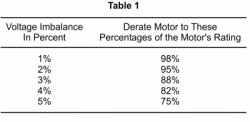DIGITAL PHASE CONVERTERS • THREE-PHASE MOTORS • ROTARY PHASE CONVERTERS • STATIC PHASE CONVERTERS • BUCK/BOOST TRANSFORMERS

 Phase Converters & Power Factor Phase Converter Efficiency Installing a Phase Converter Rotary Phase Converters Static Phase Converters VFDs as Phase Converters      • Harmonic Distortion Three-Phase Motors Phase Converters & Voltage Balance Phase Converter Applications      • Submersible Pumps      • Woodworking Equipment      • Dual Lift Stations      • Phase Converters & Welders      • Phase Converters & CNC Machines      • Phase Converters & Air Compressors      • Phase Converters & Elevators      • Phase Converters & Wire EDM      • Phase Converters & HVAC Phase Converters & Transformers      • Step-up Transformers      • Buck-Boost Transformers      • Isolation Transformers Phase Converter Experts Digital Phase Converters Regenerative Power Three-Phase Power      • Delta vs. Wye Configured Power Motor Starting Currents

# Voltage Balance and Three-Phase MotorsWeb www.phaseconverterinfo.com

## Voltage balance is probably the single most important aspect of three-phase power quality.

Just a slight voltage imbalance can damage a motor that is operating at full capacity. Voltage balance is the Achilles heel of rotary and static converters, and even utility three-phase service can be unbalanced enough to harm three-phase equipment.  The impact of this problem is evident by the huge industry in manufacturing of devices that monitor phase balance to protect motors.

To demonstrate the impact of voltage balance on a three-phase motor, measurements were taken on a 7.5 Hp rotary converter in an actual machine shop installation. The recorded line-to-line voltages were 252 V, 244.2 V and 280.5 V, which is about a 12% imbalance in the voltages. To understand how voltage imbalance will effect a motor it is useful to first transform these line-to-line voltages into their equivalent line-to-neutral voltages, which are shown in figure 3 below. These voltages are shown in what is called a phasor diagram. The magnitude of each voltage is proportional to the length of the arrow or vector and the relative phase angle of each voltage is proportional to the angle between any two arrows. The L1-L2 voltage has been arbitrarily drawn with an absolute angle of zero degrees. If one imagines that these vectors are spinning around the center point clockwise at a rate of 60 times per second, then the value of a voltage at any particular instant in time would be the projected length of its vector onto the horizontal axis. This set of vectors, which represent unbalanced voltages, rotating clockwise, can be further separated into two balanced sets of vectors, one rotating counterclockwise and called the positive-sequence voltages, and one rotating clockwise and called the negative-sequence voltages. These are also shown in figure 1 below.

FIGURE 1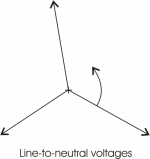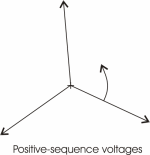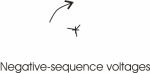An induction motor responds quite differently to the positive-sequence voltages as compared to the negative sequence voltages. The positive-sequence voltages are rotating at 60 Hz (3600 cycles/min) and if we take the example of a 2-pole motor, the rotor is spinning at about 3450 rpm. The slip speed between the field created by the positive-sequence voltages and the actual rotor velocity is low and the inductance seen by these voltages is relatively large.

If we take the example of a 7.5 Hp 240 V motor, the no-load running current is about 14 A.  The phase-to-neutral voltage is 240/(3)1/2=139 V, and the phase-to-neutral inductance is: L=139/(377*14)=26mH (1 mH=1 Henry/1000, a Henry being the basic unit of inductance).  The negative-sequence voltages are rotating in the opposite direction to the rotor velocity and the slip is almost twice the rotor velocity.  This sequence of voltages sees an inductance nearly equal to that which would be measured if the rotor were locked in position.  The locked-rotor inductance is about one sixth of the normal running inductance or about 4.4 mH for a 7.5 Hp motor.  The figure below shows the individual positive and negative sequence currents and the combined current for the voltages shown above.

FIGURE 2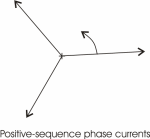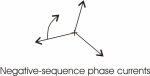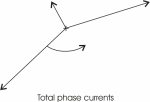Notice that while the voltages were only 12% out of balance, the currents differ by almost a factor of three.  Since the negative sequence voltages feed into an inductance which is one sixth of the inductance seen by the positive-sequence voltages, a rather modest imbalance in the voltages produces a totally unacceptable imbalance in the currents.

In this example, the lead to the motor carrying the smallest current could be totally disconnected and it would not significantly change the performance of the motor.

If a single motor is always run at a constant load, and the rotary phase converter and its associated capacitor bank are carefully adjusted, then it is possible to achieve better than a 12% voltage imbalance as discussed in the example above and get acceptable operation of the motor. The procedure would involve setting up the system of phase converter, motor and load; then measuring the generated voltages and the currents in each motor phase. If the current balance were unacceptable, then capacitors would need to be either added to, or taken out of the capacitor bank until the currents were balanced. In some cases, it might be necessary to switch to a different size phase converter to get the system balanced.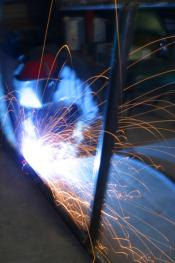If the motor were required to operate over a wide range of load conditions, or if several motors were powered using the same phase converter, it would be nearly impossible to get good voltage balance over the whole range of operation. If none of the motors were run at their full capacity, the job of getting everything to work properly would be easier. If the motor(s) were run at their full capacity for extended periods, such as in pumping applications, they would not tolerate voltage imbalance.

In summary, phase imbalance adversely impacts both the performance and the life of a motor. Even modest voltage imbalance between the phases will require a motor to be de-rated as indicated in Table 1. Phase imbalance will significantly reduce the life of motors that have a high duty cycle and operate at their maximum rated capacity.